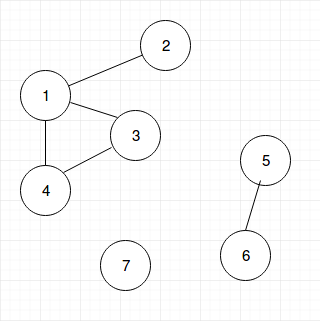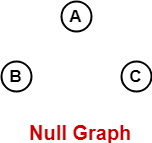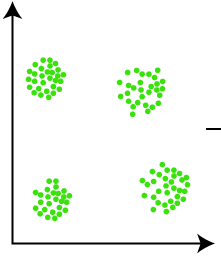• Feedback: We are listening to your every feedback, and taking action to constantly improve your learning experience. If you have any feedback, please use this form: https://thealgorists.com/Feedback.

A connected component of an undirected graph is a maximal set of nodes such that each pair of nodes is connected by a path. What I mean by this is: a connected component of an undirected graph is a subgraph in which any two vertices are connected to each other by path(s), and which is connected to no additional vertices in the rest of the graph outside the subgraph. For example, the graph shown in the illustration has three connected components.A vertex with no edges is itself a connected component.
A graph that has all the edges reachable from each other is itself connected and has exactly one connected component, consisting of the whole graph.

• The concept of Connected Components is only applicable to Undirected Graphs. The equivalent concept for Directed Graph is Strongly Connected Components .

You would have easy time understanding the logic behind how to find Connected Components if you already understand how DFS (Depth First Search) really well.

Notice from the image above how if you start DFS (Depth First Search) from any node belonging to a connected component, you would be able to discover all the nodes in the connected component.

So the algorithm to find all connected components in a given graph is simple: for every unvisited node in a given graph, do a DFS (Depth First Search) on that node, and by the time the Depth First Search is done on all the nodes in the graph all the connected components in the graph would be discovered. The simple well-commented code below shows how to implement this algorithm efficiently.

Java code:

Python code:

#### Time Complexity:

It's the DFS (Depth First Search) that does all the job to find Connected Components. The time complexity of finding Connected Components would be same as that of Depth First Search, if the code is implemented efficiently so that there is no additional overhead in the implementation to worsen the time complexity. So the time complexity of finding all Connected Components in a graph is :
• O(|V| + |E|), if the graph is implemented using Adjacency List.
|V| = total number of vertices in the graph,
|E| = total number of edges in the given graph.

If your graph is implemented using adjacency lists, wherein each node maintains a list of all its adjacent edges, then, for each node, you could discover all its neighbors by traversing its adjacency list just once in linear time. For a directed graph, the sum of the sizes of the adjacency lists of all the nodes is E (total number of edges). So, the complexity of DFS is O(V) + O(E) = O(V + E).

In best case: the given graph would have a null graph with no edges. So O(|V| + |E|) = O(|V| + |1|) = O(|V|)In average case, the given graph is moderately sparse. So, O(|E|) = O(|V|).
Therefore, O(|V| + |E|) = O(|V| + |V|) = O(|V|)

In worst case, given graph is dense. So, O(|E|) = O(|V2|) .
Therefore, O(|V| + |E|) = O(|V| + |V2|) = O(|V2|) .

• O(|V|2), if the graph is implemented using Adjacency Matrix.

If your graph is implemented as an adjacency matrix (a V x V array), then, for each node, you have to traverse an entire row of length V in the matrix to discover all its outgoing edges. Please note that each row in an adjacency matrix corresponds to a node in the graph, and the said row stores information about edges stemming from the node. So, the complexity of DFS is O(V * V) = O(V^2).

``` This is how I like to think about Connected Components: You can think each of the Connected Components to consist of items which are similar to each other. People with Data Mining background might find the concept of Connected Components similar to the concept of Clusters.Connected Components are each like cluster of similar objects. So, if you think you could design a solution for a problem by doing grouping of items based on some criteria (let's call it, similarity factor) then there is high chance that the problem could be solved by Connected Components. ```

Now let's solve a fun little problem to have a very good idea of how the concept of Connected Components could be used to solve real-world problems.

### Merge Intervals:

Given an array of intervals where intervals[i] = [starti, endi], merge all overlapping intervals, and return an array of the non-overlapping intervals that cover all the intervals in the input.

Example 1:
Input: intervals = [[1,3],[2,6],[8,10],[15,18]]
Output: [[1,6],[8,10],[15,18]]
Explanation: Since intervals [1,3] and [2,6] overlaps, merge them into [1,6].

Example 2:
Input: intervals = [[1,4],[4,5]]
Output: [[1,5]]
Explanation: Intervals [1,4] and [4,5] are considered overlapping.

#### Solution:

This problem could be solved in various different ways. If you have a strong understanding of the concept and applications of Connected Components, you would be very easily be able to think of the below solution.

What we are interested here is to find the overlapping intervals and then merge them. So if we form a graph where all the overlapping intervals would be connected to each other (i.e, add an undirected edge between interval A and interval B iff interval A and interval B are overlapping) then the overlapping intervals would form Connected Component. Now all we need to do is: for each connected component merge all the intervals in the connected component into one. The code below shows how this could be efficiently implemented.

#### The above content is written by: